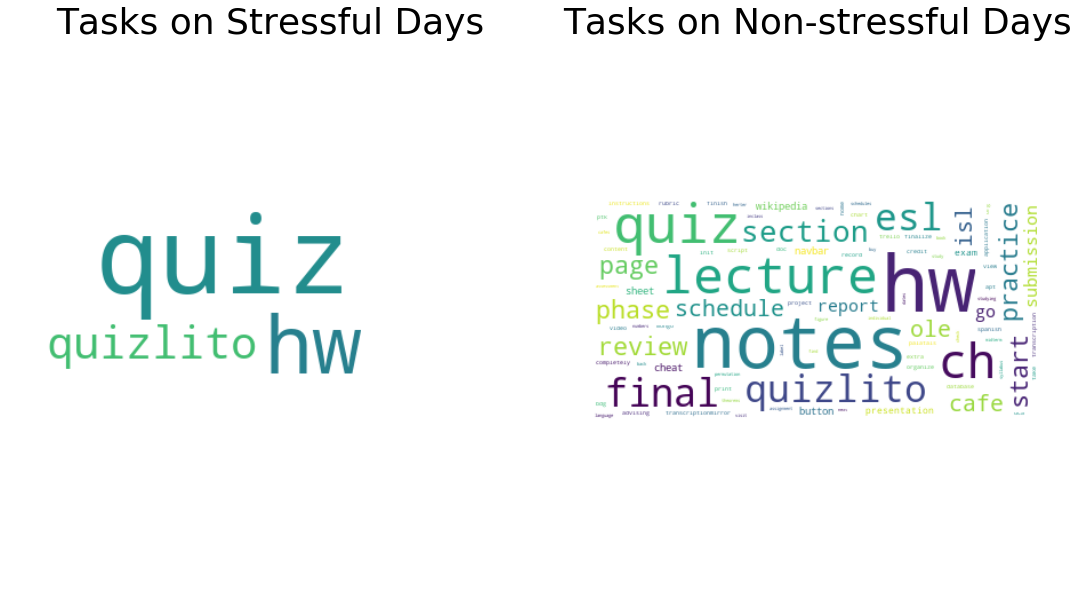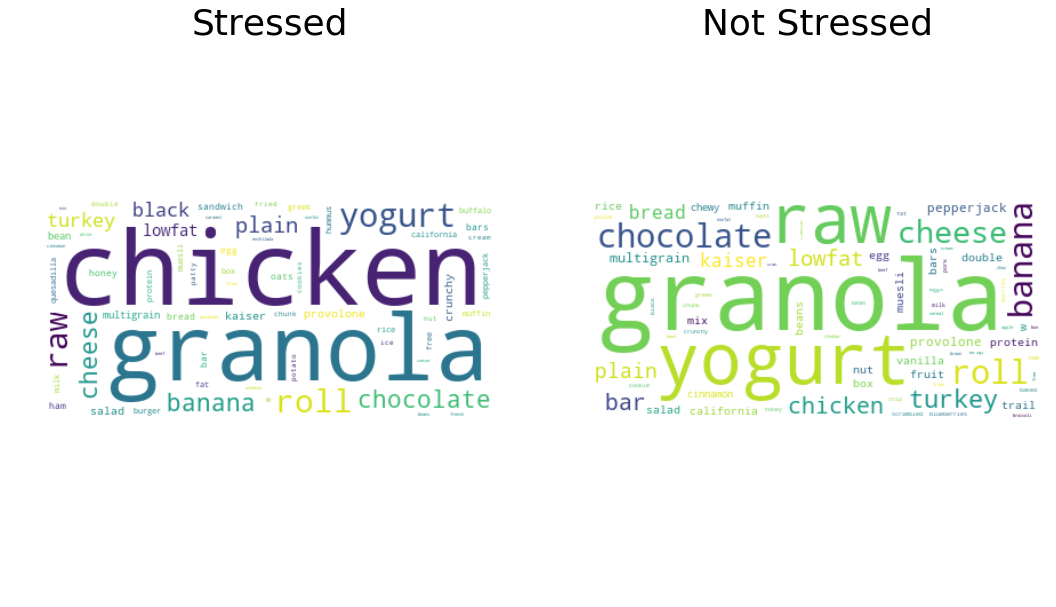Data Visualization

## Tracking Stress at University Part 2 - A Visual Summary

During my winter break, I contemplated a lot about my previous two semesters at university. I had been stressed constantly and I frequently overworked myself leading to more stress. Before I started Spring 2019, I devised a project that I could use to track and hopefully mitigate my stress while also flexing my data science muscles a bit. In this blog post I made on the first day of the semester, I detailed the variables that I would be tracking and the analyses I thought might be interesting.

However, I believe I might have inadvertantly become a victim of Goodhart’s law, best phrased by Marilyn Strathern: “When a measure becomes a target, it ceases to be a good measure.” Throughout the semester, my stress levels were significantly lower, my classes required less work, and overall, I felt as though I was in a much better place. Now, make no mistake, this is great news for me personally. However, the visualizations aren’t as cool as had hoped.

Either way, here I present the visualizations that I tracked during my semester in order to keep my mental in check.

``````import datetime as dt
import pytz

import numpy as np
import pandas as pd
import json
from ics import Calendar
from lib.python_fitbit import fitbit
from lib.python_fitbit import gather_keys_oauth2 as Oauth2

import matplotlib.pyplot as plt
import plotly.graph_objs as go
from plotly import tools
from wordcloud import WordCloud

from lib import custom_utils

init_notebook_mode(connected=True)
``````

### Constants

``````START_DATE = dt.datetime.strptime('2019-01-22', '%Y-%m-%d')
END_DATE = dt.datetime.strptime('2019-05-10', '%Y-%m-%d')

local = pytz.timezone ("America/New_York")

START_DATE_UTC = local.localize(START_DATE, is_dst=None).astimezone(pytz.utc)
END_DATE_UTC = local.localize(END_DATE, is_dst=None).astimezone(pytz.utc)
``````

``````daily_log_df = pd.read_csv('data/Daily Log (Responses) - Form Responses 1.csv', parse_dates=[0, 6])

# get relevant dates
daily_log_df = daily_log_df[(daily_log_df['Timestamp'] > START_DATE) & (daily_log_df['Timestamp'] < END_DATE)]

``````
Timestamp Stress Happiness Energy Motivation Notes Date Food Coma
21 2019-01-22 20:07:08 2 8 8 8 First day of classes. I think it's gonna be go... 2019-01-22 1
22 2019-01-23 21:51:14 3 7 8 8 Had all classes now. I'm going to have to work... 2019-01-23 2
23 2019-01-24 20:13:42 3 7 8 7 Everything is still going well for keeping up ... 2019-01-24 1
24 2019-01-25 20:49:29 2 8 7 8 Chilling at the SASA party right now. It's pre... 2019-01-25 1
25 2019-01-26 20:07:14 2 7 7 6 Chilling all day. Went to the gym to start the... 2019-01-26 1

## Simple Stress Timeseries

``````stress_trace = go.Scatter(
x=daily_log_df.Date,
y=daily_log_df.Stress,
name='Stress Level',
fill='tozeroy',
)
data = [stress_trace]
layout = go.Layout(
title='Stress Level',
yaxis={
'title': 'Stress Level',
'range': [1, 8]

}
)

fig = go.Figure(data=data, layout=layout)
iplot(fig, filename='stress')
``````

# Work

How much does what I need to complete correlate with my stress?

``````asana_df = pd.read_csv('data/asana-umass-s19.csv', parse_dates=[1, 2, 3, 8, 9])
``````
0 1120800788569533 2019-05-01 2019-05-04 2019-05-04 final report Spanish 306 NaN NaN NaT 2019-05-04 NaN NaN UMass NaN
1 1120292396473715 2019-04-28 2019-04-30 2019-04-30 final submission CS 326 NaN NaN NaT 2019-04-30 NaN NaN UMass NaN
2 1120002043175716 2019-04-25 2019-04-25 2019-04-25 go to wikipedia page button CS 326 NaN NaN NaT 2019-04-25 NaN NaN UMass NaN
3 884979504873319 2019-04-24 2019-05-02 2019-05-02 Final cheat sheet Stats 516 NaN NaN NaT 2019-05-01 NaN NaN UMass NaN
4 1119773356459372 2019-04-23 2019-04-30 2019-04-30 hw 5 CS 589 NaN NaN NaT 2019-05-02 NaN NaN UMass NaN

## Due Dates vs Stress

``````due_date_val_counts = asana_df['Due Date'].value_counts()

due_date_freqs = []
for i in range((END_DATE - START_DATE).days + 1):
date_str = (START_DATE + dt.timedelta(days=i)).strftime('%Y-%m-%d')
date_val = due_date_val_counts.get(date_str, 0)
num = date_val.values if len(date_val)>0 else 0
due_date_freqs.append({
'date': date_str,
'num': num
})
due_date_freqs_df = pd.DataFrame(due_date_freqs)
``````
``````due_date_trace = go.Bar(
x=due_date_freqs_df.date,
y=due_date_freqs_df.num,
name='Due Dates',
yaxis='y2'
)

data = [stress_trace, due_date_trace]
layout = go.Layout(
title='Stress Level vs Number of Due Dates',
yaxis1=dict(
title='Stress Level',
overlaying='y2',
range=[0, 8]
),
yaxis2=dict(
title='Due Dates',
side='right',
range=[0, 8]
),
)

fig = go.Figure(data=data, layout=layout)
iplot(fig, filename='stress-vs-due-dates')
``````

## Wordcloud of Due Dates on Stressful vs Non-stressful Days

``````stressful_dates = daily_log_df[daily_log_df.Stress > 3].Date
nonstressful_dates = daily_log_df[daily_log_df.Stress <= 3].Date
``````
``````stress_tasks = asana_df[asana_df['Due Date'].isin(stressful_dates)]
``````
``````# concatenate all name fields from tasks separated by duration of 3 days

# prep text
stress_wordcloud = custom_utils.generate_wordcloud(stress_text)
nonstress_wordcloud = custom_utils.generate_wordcloud(nonstress_text)

# display wordclouds using matplotlib
f, axes = plt.subplots(1, 2, sharex=True)
f.set_size_inches(18, 10)
axes.imshow(stress_wordcloud, interpolation="bilinear")
axes.axis("off")
axes.imshow(nonstress_wordcloud, interpolation="bilinear")
axes.axis("off")
``````
``````(-0.5, 399.5, 199.5, -0.5)
``````## Number of Incomplete Tasks vs Stress

``````incomplete_task_counts = []
for i in range((END_DATE - START_DATE).days + 1):
date = START_DATE + dt.timedelta(days=i)
'date': date,
'num': len(asana_df[(asana_df['Created At'] <= date) & ((asana_df['Completed At'] >= date) | (asana_df['Completed At'].isnull()))].index)
})
``````
``````incomplete_tasks_trace = go.Bar(
yaxis='y2'
)

layout = go.Layout(
title='Stress Level vs Number of Incomplete Tasks',
yaxis1=dict(
title='Stress Level',
overlaying='y2',
range=[0, 8]
),
yaxis2=dict(
side='right',
range=[0, 16]
),
)

fig = go.Figure(data=data, layout=layout)
iplot(fig, filename='rhr-vs-due-dates')
``````

## Exams vs Stress

``````with open('data/Exams_2alvmakoou6sa9ks0roaq79nic@group.calendar.google.com.ics', 'r') as f:
``````
``````exam_counts = []
for i in range((END_DATE_UTC - START_DATE_UTC).days + 1):
date = START_DATE_UTC + dt.timedelta(days=i)
num = 0
for event in exams_cal.events:
if (event.begin - date).days == 0:
num += 1

exam_counts.append({
'date': date.strftime('%Y-%m-%d'),
'num': num
})

exam_counts = pd.DataFrame(exam_counts)
``````
``````exams_trace = go.Bar(
x=exam_counts.date,
y=exam_counts.num,
name='Exams',
yaxis='y2'
)

data = [stress_trace, exams_trace]
layout = go.Layout(
title='Stress Level vs Exams',
yaxis1=dict(
title='Stress Level',
range=[0, 8]
),
yaxis2=dict(
title='Exams',
overlaying='y',
side='right',
range=[0, 2]
),
)

fig = go.Figure(data=data, layout=layout)
iplot(fig, filename='stress-vs-exams')
``````

# Body

How does my body respond to stress?

### Setup Fitbit API Client

``````with open('keys.json', 'r') as f:

server = Oauth2.OAuth2Server(keys['fitbit_client_id'], keys['fitbit_client_secret'])
server.browser_authorize()
ACCESS_TOKEN = str(server.fitbit.client.session.token['access_token'])
REFRESH_TOKEN = str(server.fitbit.client.session.token['refresh_token'])
fitbit_client = fitbit.Fitbit(keys['fitbit_client_id'], keys['fitbit_client_secret'], oauth2=True, access_token=ACCESS_TOKEN, refresh_token=REFRESH_TOKEN)
``````
``````[12/Jun/2019:19:22:39] ENGINE Listening for SIGTERM.
[12/Jun/2019:19:22:39] ENGINE Listening for SIGHUP.
[12/Jun/2019:19:22:39] ENGINE Listening for SIGUSR1.
[12/Jun/2019:19:22:39] ENGINE Bus STARTING
CherryPy Checker:
The Application mounted at '' has an empty config.

[12/Jun/2019:19:22:39] ENGINE Serving on http://127.0.0.1:8080
[12/Jun/2019:19:22:39] ENGINE Bus STARTED

127.0.0.1 - - [12/Jun/2019:19:22:41] "GET /?code=6ca26bec02cd3e194d7a3d292acd43579d406bdf&state=yWAKtBkwQV1kPMpOPi1dePViirgWh3 HTTP/1.1" 200 122 "" "Mozilla/5.0 (X11; Linux x86_64) AppleWebKit/537.36 (KHTML, like Gecko) Ubuntu Chromium/74.0.3729.169 Chrome/74.0.3729.169 Safari/537.36"

[12/Jun/2019:19:22:42] ENGINE Bus STOPPING
[12/Jun/2019:19:22:47] ENGINE HTTP Server cherrypy._cpwsgi_server.CPWSGIServer(('127.0.0.1', 8080)) shut down
[12/Jun/2019:19:22:47] ENGINE Bus STOPPED
[12/Jun/2019:19:22:47] ENGINE Bus EXITING
[12/Jun/2019:19:22:47] ENGINE Bus EXITED
[12/Jun/2019:19:22:47] ENGINE Waiting for child threads to terminate...
``````

## Resting Heart Rate vs Stress

``````heart_ts = fitbit_client.time_series('activities/heart',
base_date=START_DATE.strftime('%Y-%m-%d'),
end_date=END_DATE.strftime('%Y-%m-%d'))

rhr_data = []
for row in heart_ts['activities-heart']:
try:
restingHeartRate = row['value']['restingHeartRate']
except:
restingHeartRate = restingHeartRate

rhr_data.append({
'date': row['dateTime'],
'rhr': restingHeartRate
})
rhr_df = pd.DataFrame(rhr_data)
``````
``````rhr_trace = go.Scatter(
x=rhr_df.date,
y=rhr_df.rhr,
name='Resting Heart Rate',
yaxis='y2',
fill='tozeroy',
)
data = [stress_trace, rhr_trace]
layout = go.Layout(
title='Stress Level vs Resting Heart Rate',
yaxis=dict(
title='Stress Level',
range=[0, 8]
),
yaxis2=dict(
title='Resting Heart Rate',
overlaying='y',
side='right',
range=[45, 70]
)
)

fig = go.Figure(data=data, layout=layout)
iplot(fig, filename='stress-vs-rhr')
``````

## Sleep vs Stress

The missing data in mid-May is because my Fitbit ran out of battery over spring break and I forgot to bring my charger.

``````sleep_logs = []
for i in range((END_DATE - START_DATE).days + 1):
try:
date_str = (START_DATE + dt.timedelta(days=i))
sleep_log = fitbit_client.get_sleep(date=date_str)
sleep_logs.append({
'date': date_str,
'deep': sleep_log['summary']['stages']['deep'] / 60,
'light': sleep_log['summary']['stages']['light']  / 60,
'rem': sleep_log['summary']['stages']['rem']  / 60,
'wake': sleep_log['summary']['stages']['wake']  / 60,
'total': sleep_log['summary']['totalMinutesAsleep'] / 60
})
except Exception as e:
print(e)
sleep_df = pd.DataFrame(sleep_logs)
``````
``````'stages'
'stages'
'stages'
'stages'
'stages'
'stages'
'stages'
``````
``````sleep_trace = go.Bar(
x=sleep_df.date,
y=sleep_df.total,
name='Sleep',
yaxis='y2'
)

data = [stress_trace, sleep_trace]
layout = go.Layout(
title='Stress Level vs Sleep',
barmode='stack',
yaxis=dict(
title='Stress Level',
overlaying='y2',
range=[0, 8]
),
yaxis2=dict(
title='Sleep (Hrs)',
side='right',
range=[0, 10]
)
)

fig = go.Figure(data=data, layout=layout)
iplot(fig, filename='stress-vs-sleep')
``````

## Sleep Stages before Stressful vs Non-stressful Days

``````stress_sleep_logs = sleep_df[sleep_df['date'].isin(stressful_dates)]
nonstress_sleep_logs = sleep_df[sleep_df['date'].isin(nonstressful_dates)]

stress_sleep_sums = stress_sleep_logs.sum()
nonstress_sleep_sums = nonstress_sleep_logs.sum()
``````
``````fig = {
"data": [
{
"labels": stress_sleep_sums.keys(),
"values": stress_sleep_sums.values,
"domain": {"x": [0, .48]},
"name": "Stressful Sleep Stages",
"hoverinfo":"label+percent+name",
"hole": .4,
"type": "pie"
},
{
"labels": nonstress_sleep_sums.keys(),
"values": nonstress_sleep_sums.values,
"domain": {"x": [.52, 1]},
"name": "Non-stressful Sleep Stages",
"hoverinfo":"label+percent+name",
"hole": .4,
"type": "pie"
}
],
"layout": {
"title": "Sleep Stages before Stressful vs Non-stressful Days",
"annotations": [
{
"font": {
"size": 20
},
"showarrow": False,
"text": "Stress",
"x": 0.20,
"y": 0.5
},
{
"font": {
"size": 20
},
"showarrow": False,
"text": "Non-stress",
"x": 0.83,
"y": 0.5
}
]
}
}
iplot(fig, filename='donut')
``````

# Habits

How do my actions change when I’m stressed?

## Caloric Intake vs Stress

``````meal_type = { 1: 'Breakfast', 2: 'Morning Snack', 3: 'Lunch', 4: 'Afternoon Snack', 5: 'Dinner', 7: 'Anytime' }
cals_per_nutrient = { 'carbs': 4, 'fat': 9, 'protein': 4 }
``````
``````macronutrient_logs = []
for i in range((END_DATE - START_DATE).days + 1):
date = START_DATE + dt.timedelta(days=i)
food_log = fitbit_client.foods_log(date=date)
macronutrient_logs.append({
'date': date,
'cals_from_carbs': food_log['summary']['carbs']*cals_per_nutrient['carbs'],
'cals_from_fat': food_log['summary']['fat']*cals_per_nutrient['fat'],
'cals_from_protein': food_log['summary']['protein']*cals_per_nutrient['protein'],
'total_cals': food_log['summary']['calories'],
'foods_eaten': [item['loggedFood']['name'] for item in food_log['foods']]
})
macronutrient_df = pd.DataFrame(macronutrient_logs)
``````
``````cals_trace = go.Bar(
x=macronutrient_df.date,
y=macronutrient_df.total_cals,
name='Calories',
yaxis='y2'
)

data = [stress_trace, cals_trace]
layout = go.Layout(
title='Stress Level vs Caloric Intake',
barmode='stack',
yaxis=dict(
title='Stress Level',
overlaying='y2',
range=[0, 8]
),
yaxis2=dict(
title='Calories',
side='right',
range=[0, 4000]
)
)

fig = go.Figure(data=data, layout=layout)
iplot(fig, filename='stacked-bar')
``````

## Carbs, Fat, Protein Avg on Stressful vs Non-stressful Days

``````stress_food_logs = macronutrient_df[macronutrient_df['date'].isin(stressful_dates)]
nonstress_food_logs = macronutrient_df[macronutrient_df['date'].isin(nonstressful_dates)]

stress_food_sums = stress_food_logs.sum()
nonstress_food_sums = nonstress_food_logs.sum()
``````
``````fig = {
"data": [
{
"labels": stress_food_sums.keys(),
"values": stress_food_sums.values,
"domain": {"x": [0, .48]},
"name": "Stressful Caloric Intake",
"hoverinfo":"label+percent",
"hole": .4,
"type": "pie"
},
{
"labels": nonstress_food_sums.keys(),
"values": nonstress_food_sums.values,
"domain": {"x": [.52, 1]},
"name": "Non-stressful Caloric Intake",
"hoverinfo":"label+percent",
"hole": .4,
"type": "pie"
}
],
"layout": {
"title": "Caloric Intake on Stressful vs Non-stressful Days",
"annotations": [
{
"font": {
"size": 20
},
"showarrow": False,
"text": "Stress",
"x": 0.20,
"y": 0.5
},
{
"font": {
"size": 20
},
"showarrow": False,
"text": "Non-stress",
"x": 0.83,
"y": 0.5
}
]
}
}
iplot(fig, filename='donut')
``````

## Wordcloud of Foods Eaten on Stressful vs Non-stressful Days

``````stress_food_str = ' '.join([' '.join(foods_for_day) for foods_for_day in stress_food_logs['foods_eaten'].values])
nonstress_food_str = ' '.join([' '.join(foods_for_day) for foods_for_day in nonstress_food_logs['foods_eaten'].values])
``````
``````# prep text
stress_wordcloud = custom_utils.generate_wordcloud(stress_food_str)
nonstress_wordcloud = custom_utils.generate_wordcloud(nonstress_food_str)

# display wordclouds using matplotlib
f, axes = plt.subplots(1, 2, sharex=True)
f.set_size_inches(18, 10)
axes.imshow(stress_wordcloud, interpolation="bilinear")
axes.set_title('Stressed', fontsize=36)
axes.axis("off")
axes.imshow(nonstress_wordcloud, interpolation="bilinear")
axes.set_title('Not Stressed', fontsize=36)
axes.axis("off")
``````
``````(-0.5, 399.5, 199.5, -0.5)
``````## Top 10 Website Usage on Stressful vs Non-stressful Days

``````webtracker_df = pd.read_csv('data/webtime-tracker.csv', index_col=0).transpose()
``````
Domain 0.0.0.0 1.1.1.1 127.0.0.1 192.168.1.1 192.168.123.1 2.bp.blogspot.com 2ality.com 326webprojectslack.slack.com 4.bp.blogspot.com 66.media.tumblr.com ... www8.garmin.com xarray.pydata.org yalebooks.yale.edu yourbasic.org youthful-sage.glitch.me youtu.be yutsumura.com z-table.com zellwk.com zulko.github.io
2018-11-22 0 0 0 0 0 0 0 0 0 0 ... 0 0 0 0 0 0 0 0 0 0
2018-11-22.1 0 0 0 0 0 0 0 0 0 0 ... 0 0 0 0 0 0 0 0 0 0
2018-11-23 0 0 0 0 0 0 0 0 0 0 ... 0 0 0 0 0 0 0 0 0 0
2018-11-24 0 0 0 0 0 0 0 0 0 0 ... 0 0 0 0 0 0 0 0 0 0
2018-11-25 0 0 0 0 0 0 0 0 0 0 ... 0 0 0 0 0 0 0 0 0 0

5 rows × 1183 columns

``````stressful_date_strs = list(stressful_dates.apply(lambda x: x.strftime('%Y-%m-%d')))
nonstressful_date_strs = list(nonstressful_dates.apply(lambda x: x.strftime('%Y-%m-%d')))

stress_domains = webtracker_df.loc[stressful_date_strs].sum(axis=0).sort_values(ascending=False)
nonstress_domains = webtracker_df.loc[nonstressful_date_strs].sum(axis=0).sort_values(ascending=False)
``````
``````fig = {
"data": [
{
"labels": list(stress_domains[:10].keys()) + ['Other'],
"values": list(stress_domains[:10].values) + [stress_domains[10:].sum()],
"domain": {"x": [0, .48]},
"name": "Stressful Surfing",
"hoverinfo":"label+percent",
"hole": .4,
"type": "pie"
},
{
"labels": list(nonstress_domains[:10].keys()) + ['Other'],
"values": list(nonstress_domains[:10].values) + [nonstress_domains[10:].sum()],
"domain": {"x": [.52, 1]},
"name": "Non-stressful Surfing",
"hoverinfo":"label+percent",
"hole": .4,
"type": "pie"
}
],
"layout": {
"title": "Website Usage on Stressful vs Non-stressful Days",
"annotations": [
{
"font": {
"size": 20
},
"showarrow": False,
"text": "Stress",
"x": 0.19,
"y": 0.5
},
{
"font": {
"size": 20
},
"showarrow": False,
"text": "Non-stress",
"x": 0.85,
"y": 0.5
}
]
}
}
iplot(fig, filename='donut')
``````

## Stress Level vs Minutes Sedentary

``````sed_ts = fitbit_client.time_series('activities/minutesSedentary',
base_date=START_DATE.strftime('%Y-%m-%d'),
end_date=END_DATE.strftime('%Y-%m-%d'))

sed_data = []
for row in sed_ts['activities-minutesSedentary']:
sed_data.append({
'date': row['dateTime'],
'rhr': row['value']
})
sed_df = pd.DataFrame(sed_data)
``````
``````sedentary_trace = go.Scatter(
x=sed_df.date,
y=sed_df.rhr,
name='Minutes Sedentary',
yaxis='y2',
fill='tozeroy',
)
data = [stress_trace, sedentary_trace]
layout = go.Layout(
title='Stress Level vs Minutes Sedentary',
yaxis=dict(
title='Stress Level',
range=[0, 8]
),
yaxis2=dict(
title='Minutes Sedentary',
overlaying='y',
side='right',s
range=[0, 1600]
)
)

fig = go.Figure(data=data, layout=layout)
iplot(fig, filename='stress-vs-min-sedentary')
``````